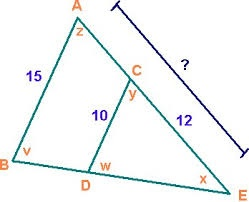# Triangles 6682

Triangles ABC and A'B'C'. They are similar. In triangle ABC, the measures of two angles are 25 degrees and 65 degrees.
Explain why in triangle A'B'C' the sum of the sizes of the two angles is equal to 90 degrees

x =  90 °

### Step-by-step explanation:Did you find an error or inaccuracy? Feel free to write us. Thank you!

Tips for related online calculators